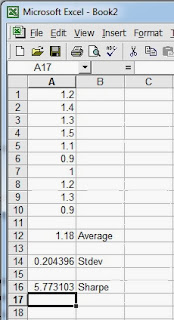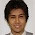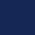## Pages

### Sharpe Ratio for Betting

David Edelman's The Compleat Horseplayer gives a betting equivalent for the Sharpe Ratio (pages 27 & 28). The Sharpe Ratio (aka Information Ratio) is used as a measure of effectiveness for a strategy.

In finance, the Sharpe Ratio is defined "as the excess return (or risk premium) per unit of deviation in an investment asset or a trading strategy, typically referred to as risk (and is a deviation risk measure), named after William Forsyth Sharpe." The ratio measures how well the return of an asset compensates the investor for the risk taken.

In sports betting the Sharpe Ratio is a measure of how much a trader is rewarded for any particular system they devise.The ratio is given by the excess returns divided by standard deviation (aka volatility) and can be computed in Excel with the formula

=average(a1:a10) / stdev(a1:a10)

where a1:10 is your array of returns from any single strategy.

To the right you can see a sample of returns for which I have created a Sharpe Ratio. Play around with the returns (percentage or absolute) in cells A1 to A10. Put in some negative values to denote losses and also widely differing positive and negative returns to get a feel for what the Sharpe Ratio measures.

For example, you only have to change one of the values to -1.0 and the standard deviation will increase thus dramatically lowering the ratio.

In finance a good ratio is regarded as being greater than 2. In other words, your average return for any trading strategy should be twice that of the standard deviation of the returns. A Sharpe Ratio of 1 means that the return is equal to the risk being taken and less than 1 means that more risk is being taken than the reward in return. The higher the Sharpe Ratio then the lower the deviation of returns compared with the average. In other words, no sudden big losses that can wipe out your bankroll. Slow and steady wins the race.

For sports trading I have tried to calculate a Sharpe Ratio after every trade but that produces very low figures. Instead, I calculate a figure for total yield at the end of the day and produce a Sharpe Ratio based on daily yields. Generally, the longer the period the larger the ratio. You could calculate a weekly or monthly yield and base your ratio on that. However, if your trading models are short lived then the daily ratio is probably the best.

I use Sharpe Ratio as one of many fitness measures when developing new trading models. See GA Optimisation of Entries and Exits.

1.Hi, first of all congratulations for your blog. What would be the volatility? I dont get how to enter the formula. I can calculate the Roi, is it something to fo with liability? Thanks a lot for the info. Guido

2.I have updated the article and hope that you understand it better.

3.Great, thanks a lot! I understand it better now. I'm actually testing a laying strategy that gave me 30% ROI on 53 selections (over 500 races) so far, now Im putting my money in it and wanted to measure all the risks involved.
All my positive returns are 0,95 (laying).
I get an average of 0,52 (with losses included of course), the stdev is 1.04, so (0,52/1,04=0,49) my sharpe ratio is 0,49. Does it sounds good to you? Am I doing the calculation right? Thanks again for your time, Im reading all your articles, there is very informative and serious stuff!!

4.Let me see. So you made a spreadsheet with 53 returns in it. The average return was 0.52 and the stdev was 1.04 giving a ratio of 0.49

From experience I would say that is a winning strategy but one that could suffer a major downturn that could wipe out your bankroll.

Have you done anything with Monte Carlo simulation and simulated your strategy a couple of million times? That could tell you the probability of a bankroll eating event.

5.Today I've tried to figure out a way of doing the montecarlo simulation on excel reading several manuals but I've found it extremely maybe due to my very basic level of mathematics.
I will keep on searching that.

6.For something like this I would program it in a separate application. A simple Excel syle spreadsheet would be a laborious task to create for something like this. My Martingale sim for roulette was a little basic but all that was needed to prove my point.

If you are loath to learn programming then there might be an add-on Excel package to buy somewhere. That would contain VBA code to handle the simulation.

Have a look at http://xlsim.com/xlsim/index.html

I would not be afraid to learn programming, you would learn mathematics at the same time. And the more maths you learn the more understanding you will get of betting markets.

7.Hi James,

Let me give an example:
- 10 races
- 9 greens, \$30 each one
- 1 red, -\$10 each one

So I will get:
- \$26 average
- 2.06 sharp ratio

So winning 90% of time with greens 3x more than red I can get the "good ratio" that you said (greater than 2).

Looks like it's difficult to get a "really good ratio"!

Is it right?

1.The maths is correct. However, the example above is simplistic. You would want a much larger sample.

And yes, getting a good ratio is hard and I often settle for one between 1.5 and 2.0

The figure of 2.0 is from the financial world.

2.Ok! I see. Thank you so much.

It is interesting to see you keep writing and replying comments here... looks like you really like what you do.

Ok, I'm reading all your posts making notes and checking the books... but what should I do first? Maybe the book "Inside the black box"?

3.Yes, I do enjoy me work, very much.

By quant trading, are you referring to sports or financials?

Inside the Black Box is a good one for learning black box trading and how quants approach algorithmic trading.

8.Hi James,

Aren't you missing one of the Sharpe Ratio's components, the risk-free return? Is it not necessary for our purposes to use that benchmark?

Thank you.

1.The financial version of the Sharpe Ratio does utilise a risk-free return but what would be a risk-free return for a bettor?

A bank account or not betting at all? Either way, it is close to zero.

I have seen no version of a sports bettting Sharpe Ratio utilising a risk-free return benchmark.

2.Gotcha. Thank you for the rapid response.

I asked the question because as I was researching the Sharpe Ratio, I came across this post (https://www.drbobsports.com/essays.cfm?p=17) from Dr. Bob, who does use a risk-free return (S&P) so I was a little confused as which method to use as the results will vary depending upon which is applied.

3.Dr Bob has gone the whole hog and used the financial Sharpe Ratio, which is not that different to the sports betting version but includes a benchmark.

What Dr Bob is saying is that you have a choice; either bet on the S&P (assuming it is risk-free during the duration of your bet - it could fall in value so maybe not that risk free - the usual benchmark would be a government bond) or bet on a sports event.

My alternative is not to bet and keep it in the bank where you get next to nothing. Actually, I keep my money in bullion and P2P lending websites but I regard that money and my sports betting money as separate entities and don't benchmark one against the other.

4.Thanks again, James. Makes sense to me.

9.Just tested one of my strategies with sharp ratio formula you kindly provided and based a very modest sample size of 16 trades over a 10 day period, I have a sharp ratio: 0.9, stdev: 1.29 and sr of 75%. Don't know whether to be worried or not??? Surely to good to be true??? With sample size so small surely has to decrease?

1.Yes, a bigger sample size is a must. Try for 100.

Ideally, you want your Sharpe at least over 1.00 but 0.9 is not bad.

Try working out a daily Sharpe by % yield for the day and see if it is greater. I would assume so.

10.Thanks James! Yield is good per trade 0.95 x my initial stake £2 after comm. But will try for the 100 as per your suggestion.

This is an automated trading strategy, interestingly trading manually my daily sharp ration based on the same sample size is 0.87 with stdev: 2.1 and with my tendency to blow up is probably reflected in the stdev figure.

Begs the question is automated trading the way forward?

1.Always the way forward. Algo-trading is impassionate trading and also allows you to scale up your income.

11.'Try working out a daily Sharpe by % yield for the day and see if it is greater. I would assume so.'

Could you possibly clarify how to do this please.

1.Calculate Sharpe on these daily yields.

I wouldn't get too wedded to Sharpe. There are many ways of calculating performance.

For example the ones in the graphics in the following

I don't have time to go into detail. It will be in the next book or you can research it yourself in the meantime.

12.Hi James,

Since we are working with percentage averages, why is the geometric mean not being used to determine our avg?

Thank you.

1.Nothing is set in stone as Sharpe is an arbitrary value and open to interpretation. You may tinker with it as you see fit.